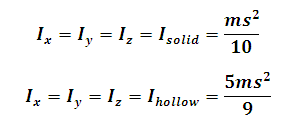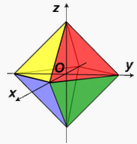# Moment of Inertia of a Octahedron Calculator

This Calctown Calculator calculates the Moment of Inertia of a Solid and Hollow Octahedron about a Perpendicular axis passing through the center.

kg
m

#### Result

kg-m2
kg-m2Click here to view image

where

Ihollow = Moment of Inertia of hollow octahedron

Isolid = Moment of Inertia of solid octahedron

m = mass of octahedron

s = side of octahedron